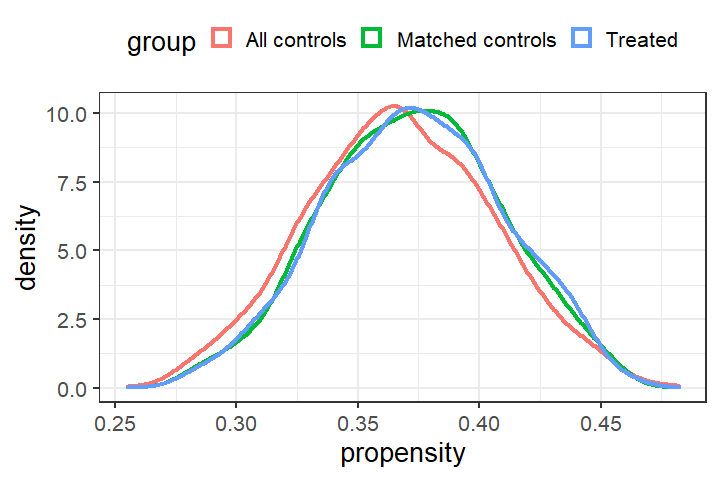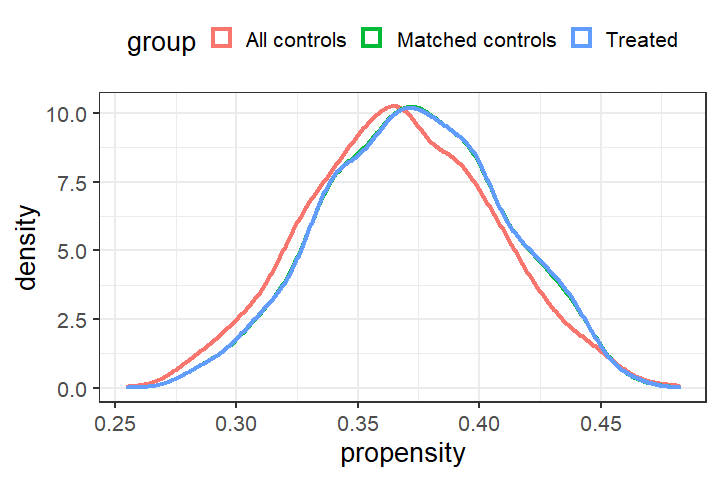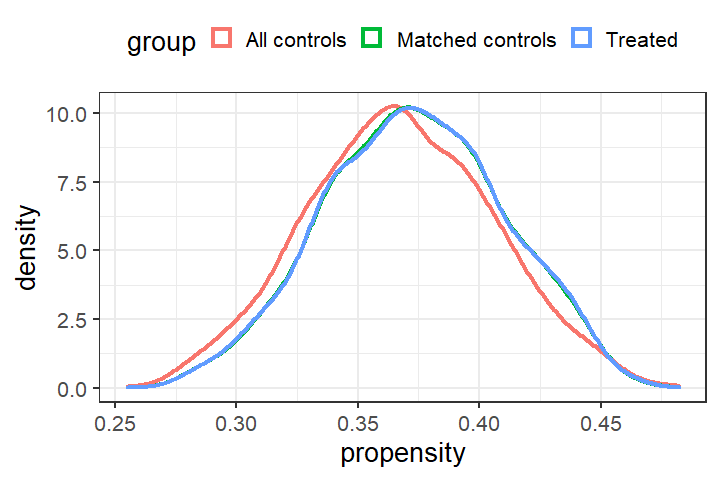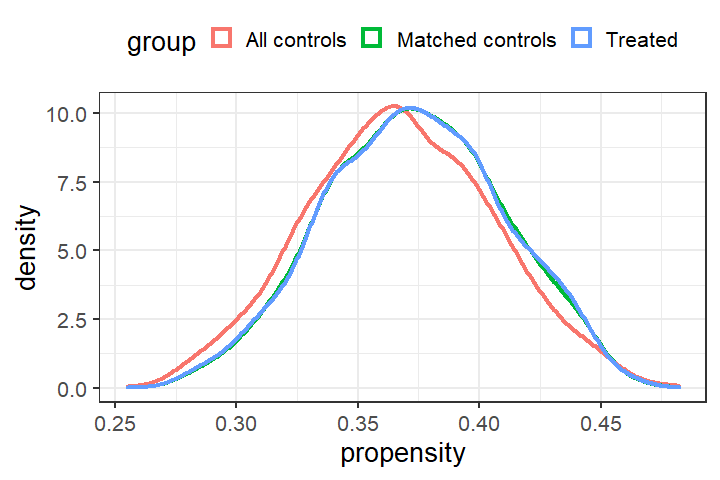# Tutorial for R Package match2C

options(scipen = 99)
options(digits = 2)
library(match2C)
library(ggplot2)
#> Warning: package 'ggplot2' was built under R version 4.0.5
library(mvtnorm)

# Introduction

## Preparation of data

This file serves as an introduction to the R package match2C. We first load the package and an illustrative dataset from Rouse (1995). For the purpose of illustration, we will mostly work with 6 covariates: two nominal (black and female), two ordinal (father’s education and mother’s education), and two continuous (family income and test score). Treatment is an instrumental-variable-defined exposure, equal to $$1$$ if the subject is doubly encouraged, meaning the both the excess travel time and excess four-year college tuition are larger than the median, and to be $$0$$ if the subject is doubly discouraged. There are $$1,122$$ subjects that are doubly encouraged (treated), and $$1,915$$ that are doubly discouraged (control).

Below, we specify covariates to be matched (X) and the exposure (Z), and fit a propensity score model.

attach(dt_Rouse)
X = cbind(female,black,bytest,dadeduc,momeduc,fincome) # covariates to be matched
Z = IV # IV-defined exposure in this dataset

# Fit a propensity score model
family=binomial)$fitted.values # Number of treated and control n_t = sum(Z) # 1,122 treated n_c = length(Z) - n_t # 1,915 control dt_Rouse$propensity = propensity
detach(dt_Rouse)

## Glossary of Matching Terms

We define some useful statistical matching terminologies:

• Bipartite Matching: Matching control subjects to treated subjects based on a binary treatment status.

• Tripartite Matching: Matching control subjects to treated subjects based on a tripartite network. A tripartite network consists of two bipartite networks: a left network and a right network, where the right network is a mirror copy of the left network in nodes, but with possibly different distance structure. Typically the left network is responsible for close pairing and the right network is responsible for balancing; See Zhang et al., (JASA, 2021) for details.

• Pair Matching: Matching one control subject to one treated subject.

• Optimal Matching: Matching control subjects to treated subjects such that some properly defined sum of total distances is minimized.

• Propensity Score: The propensity score is the conditional probability of assignment to a particular treatment given a vector of observed covariates (Rosenbaum and Rubin, 1983).

• Mahalanobis Distance: A multivariate measure of covariate distance between units in a sample (Mahalanobis, 1936). The squared Mahalanobis distance is equal to the difference in covariate values of treated units and matched control units, divided by the covariate’s standard deviation. Mahalanobis distance takes into account the correlation structure among covariates. The distance is zero if two units have the same value for all covariates and increases as two units become more dissimilar.

• Exact Matching: Matching cases to controls requiring the same value of a nominal covariate.

• Fine Balance: A matching technique that balances exactly the marginal distribution of one nominal variable or the joint distribution of several nominal variables in the treated and control groups after matching (Rosenbaum et al., 2007; Yu et al., 2020).

For more details on statistical matching and statistical inference procedures after matching, see Observational Studies (Rosenbaum, 2002) and Design of Observational Studies (Rosenbaum, 2010).

# Statistical Matching Workflow: Match, Check Balance, and (Possibly) Iterate

## An Overview of the Family of Three Matching Functions match_2C, match_2C_mat, and match_2C_list

In the package match2C, three functions are primarily responsible for the main task statistical matching. These three functions are match_2C, match_2C_mat, and match_2C_list. We will examine more closely their differences and illustrate their usage with numerous examples in later sections. In this section we give a high-level outline of what each of them does. In short, the three functions have the same output format (details in the next section), but are different in their inputs.

Function match_2C_mat takes as input at least one distance matrix. A distance matrix is a n_t-by-b_c matrix whose ij-th entry encodes a measure of distance (or similarity) between the i-th treated and the j-th control subject. Hence, function match_2C_mat is most handy for users who are familiar with constructing and working with distance matrices. One commonly-used way to construct a distance matrix is to use the function match_on in the package optmatch (Hansen, 2007).

Function match_2C_list is similar to match_2C_mat except that it requires at least one distance list as input. A list representation of a treatment-by-control distance matrix consists of the following arguments:

• start_n: a vector containing the node numbers of the start nodes of each arc in the network.
• end_n: a vector containing the node numbers of the end nodes of each arc in the network.
• d: a vector containing the integer cost of each arc in the network.

Nodes 1,2,…,n_t correspond to n_t treatment nodes, and n_t + 1, n_t + 2, …, n_t + n_c correspond to n_c control nodes. Note that start_n, end_n, and d have the same lengths, all of which equal to the number of edges. Functions create_list_from_scratch and create_list_from_mat in the package allow users to construct a (possibly sparse) distance list with a possibly user-specified distance measure. We will discuss how to construct distance lists in later sections.

Function match_2C is a wrap-up of match_2C_list with pre-specified distance list structures. For the left network, a Mahalanobis distance between covariates X is adopted; For the right network, an L-1 distance between the propensity score is used. A large penalty is applied so that the algorithm prioritizes balancing the propensity score distributions in the treated and matched control groups, followed by minimizing the sum of within-matched-pair Mahalanobis distances. Function match_2C further allows fine-balancing the joint distribution of a few key covariates. The hierarchy goes in the order of fine-balance >> propensity score distribution >> within-pair Mahalanobis distance.

## Object Returned by match_2C, match_2C_mat, and match_2C_list

Objects returned by the family of matching functions match_2C, match_2C_mat, and match_2C_list are the same in format: a list of the following three elements:

• feasible: 0/1 depending on the feasibility of the matching problem;

• data_with_matched_set_ind: a data frame that is the same as the original data frame, except that a column called matched_set and a column called distance are added to it. Variable matched_set assigns 1,2,…,n_t to each matched set, and NA to controls not matched to any treated. Variable distance records the control-to-treated distance in each matched pair, and assigns NA to all treated and controls that are left unmatched. If matching is not feasible, NULL will be returned;

• matched_data_in_order: a data frame organized in the order of matched sets and otherwise the same as data_with_matched_set_ind. Null will be returned if the matching is unfeasible.

Let’s take a look at an example output returned by the function match_2C_list. The matching problem is indeed feasible:

# Check feasibility
matching_output_example$feasible #>  1 Let’s take a look at the data frame data_with_matched_set_ind. Note that it is indeed the same as the original dataset except that a column matched_set and a column distance are appended. Observe that the first six instances belong to $$6$$ different matched sets; therefore matched_set is from $$1$$ to $$6$$. The first six instances are all treated subjects so distance is NA. # Check the original dataset with two new columns head(matching_output_example$data_with_matched_set_ind, 6)
#> 1     12     1      1     1        0     41       0       0       0       0
#> 2     14     1      1     0        0     46       0       0       0       0
#> 3     12     1      1     0        0     60       0       0       0       0
#> 4     14     1      1     0        0     61       0       0       1       0
#> 5     16     1      1     0        0     46       0       0       0       0
#> 6     12     1      0     0        0     60       0       1       0       0
#> 1    9500        0  1          0          0       0       0             1
#> 2   18000        0  1          0          0       0       0             1
#> 3   22500        0  1          1          0       1       0             4
#> 4   22500        0  1          0          0       0       2             4
#> 5       0        1  1          1          0       1       0             1
#> 6   62000        0  1          0          0       3       0             4
#>   income_quartile propensity matched_set distance
#> 1               1       0.42           1       NA
#> 2               2       0.41           2       NA
#> 3               3       0.35           3       NA
#> 4               3       0.35           4       NA
#> 5               0       0.43           5       NA
#> 6               4       0.31           6       NA

Finally, matched_data_in_order is data_with_matched_set_ind organized in the order of matched sets. Note that the first $$2$$ subjects belong to the same matched set; the next two subjects belong to the second matched set, and etc.

# Check dataframe organized in matched set indices
head(matching_output_examplematched_data_in_order, 6) #> educ86 twoyr female black hispanic bytest dadsome dadcoll momsome momcoll #> 1 12 1 1 1 0 41 0 0 0 0 #> 1779 15 0 1 1 0 42 0 0 0 0 #> 2 14 1 1 0 0 46 0 0 0 0 #> 2568 15 0 1 0 1 47 0 0 0 0 #> 3 12 1 1 0 0 60 0 0 0 0 #> 1828 16 0 1 0 0 59 0 0 0 0 #> fincome fincmiss IV dadneither momneither dadeduc momeduc test_quartile #> 1 9500 0 1 0 0 0 0 1 #> 1779 3500 0 0 1 0 1 0 1 #> 2 18000 0 1 0 0 0 0 1 #> 2568 14000 0 0 0 0 0 0 2 #> 3 22500 0 1 1 0 1 0 4 #> 1828 31500 0 0 1 0 1 0 3 #> income_quartile propensity matched_set distance #> 1 1 0.42 1 NA #> 1779 1 0.42 1 1.99 #> 2 2 0.41 2 NA #> 2568 1 0.41 2 0.29 #> 3 3 0.35 3 NA #> 1828 3 0.35 3 0.44 ## Checking Balance Statistical matching belongs to the design stage of an observational study. The ultimate goal of statistical matching is to embed observational data into an approximate randomized controlled trial and the matching process should always be conducted without access to the outcome data. Not looking at the outcome at the design stage means researchers could in principle keep adjusting their matched design until some pre-specified design goal is achieved. A rule of thumb is that the standardized differences of each covariate, i.e., difference in means after matching divided by pooled standard error before matching, is less than 0.1. Function check_balance in the package provides simple balance check and visualization. In the code chunk below, matching_output_example is an object returned by the family of matching functions match_2C_list/match_2C/match_2C_mat (we give details on how to use these functions later). Function check_balance then takes as input a vector of treatment status Z, an object returned by match_2C (or match_2C_mat or match_2C_list), a vector of covariate names for which we would like to check balance, and output a balance table. There are six columns of the balance table: 1. Mean covariate values in the treated group (Z = 1) before matching. 2. Mean covariate values in the control group (Z = 0) before matching. 3. Standardized differences before matching. 4. Mean covariate values in the treated group (Z = 1) after matching. 5. Mean covariate values in the control group (Z = 0) after matching. 6. Standardized differences after matching. tb_example = check_balance(Z, matching_output_example, cov_list = c('female', 'black', 'bytest', 'fincome', 'dadeduc', 'momeduc', 'propensity'), plot_propens = FALSE) print(tb_example) #> Z = 1 Z = 0 (Bef) Std. Diff (Bef) Z = 0 (Aft) Std. Diff (Aft) #> female 0.58 0.56 0.032 0.58 0.0000 #> black 0.19 0.18 0.011 0.18 0.0177 #> bytest 51.88 53.04 -0.098 52.23 -0.0295 #> fincome 21630.12 23439.16 -0.072 21266.04 0.0145 #> dadeduc 1.10 1.17 -0.041 1.09 0.0047 #> momeduc 0.93 1.00 -0.038 0.91 0.0181 #> propensity 0.37 0.37 0.115 0.37 0.0119 Function check_balance may also plot the distribution of the propensity score among the treated subjects, all conrol subjects, and the matched control subjects by setting option plot_propens = TRUE and supplying the option propens with estimated propensity scores as shown below. In the figure below, the blue curve corresponds to the propensity score distribution among 1,122 treated subjects, the red curve among 1,915 control subjects, and the green curve among 1,122 matched controls. It is evident that after matching, the propensity score distribution aligns better with that of the treated subjects. tb_example = check_balance(Z, matching_output_example, cov_list = c('female', 'black', 'bytest', 'fincome', 'dadeduc', 'momeduc', 'propensity'), plot_propens = TRUE, propens = propensity)# Introducing the Main Function match_2C ## A Basic Match with Minimal Input Function match_2C is a wrapper function of match_2C_list with a carefully-chosen distance structure. Compare to match_2C_list and match_2C_mat, match_2C is less flexible; however, it requires minimal input from the users’ side, works well in most cases, and therefore is of primary interest to most users. The minimal input to function match_2C is the following: 1. treatment indicator vector, 2. a matrix of covariates to be matched, 3. a vector of estimated propensity score, and 4. the original dataset to which match sets information is attached. By default, match_2C performs a statistical matching that: 1. maximally balances the marginal distribution of the propensity score in the treated and matched control group, and 2. subject to 1, minimizes the within-matched-pair Mahalanobis distances. The code chunk below displays how to perform a basic match using function match_2C with minimal input, and then check the balance of such a match. The balance is very good and the propensity score distributions in the treated and matched control group almost perfectly align with each other. # Perform a matching with minimal input matching_output = match_2C(Z = Z, X = X, propensity = propensity, dataset = dt_Rouse) tb = check_balance(Z, matching_output, cov_list = c('female', 'black', 'bytest', 'fincome', 'dadeduc', 'momeduc', 'propensity'), plot_propens = TRUE, propens = propensity)print(tb) #> Z = 1 Z = 0 (Bef) Std. Diff (Bef) Z = 0 (Aft) Std. Diff (Aft) #> female 0.58 0.56 0.032 0.58 0.0000 #> black 0.19 0.18 0.011 0.19 0.0000 #> bytest 51.88 53.04 -0.098 52.03 -0.0123 #> fincome 21630.12 23439.16 -0.072 21146.17 0.0193 #> dadeduc 1.10 1.17 -0.041 1.10 -0.0011 #> momeduc 0.93 1.00 -0.038 0.94 -0.0060 #> propensity 0.37 0.37 0.115 0.37 0.0016 ## Incorporating Exact Matching Constraints Researchers can also incorporate the exact matching constraints by specifying the variables to be exactly matched in the option exact. In the example below, we match exactly on father’s education and mother’s education. The matching algorithm still tries to find a match that maximally balance the propensity score distribution, and then minimzies the treated-to-control total distances, subject to the exact matching constraints. One can check that father’s education and mother’s education are exactly matched. Moreover, since the matching algorithm separates balancing the propensity score from exact matching, the propensity score distributions are still well balanced. # Perform a matching with minimal input matching_output_with_exact = match_2C(Z = Z, X = X, exact = c('dadeduc', 'momeduc'), propensity = propensity, dataset = dt_Rouse) # Check exact matching head(matching_output_with_exactmatched_data_in_order[, c('female', 'black', 'bytest',
'propensity', 'IV', 'matched_set')])
#>      female black bytest fincome dadeduc momeduc propensity IV matched_set
#> 1         1     1     41    9500       0       0       0.42  1           1
#> 358       1     1     39   22500       0       0       0.41  0           1
#> 2         1     0     46   18000       0       0       0.41  1           2
#> 45        1     0     46   18000       0       0       0.41  0           2
#> 3         1     0     60   22500       1       0       0.35  1           3
#> 2017      1     0     60    9500       1       0       0.37  0           3

# Check overall balance
tb = check_balance(Z, matching_output_with_exact,
cov_list = c('female', 'black', 'bytest', 'fincome', 'dadeduc', 'momeduc', 'propensity'),
plot_propens = TRUE, propens = propensity)## Incorporating Fine Balancing Constraints

Function match_2C also allows incorporating the (near-)fine balancing constraints. (Near-)fine balance refers to maximally balancing the marginal distribution of a nominal variable, or more generally the joint distribution of a few nominal variables, in the treated and matched control groups. Option fb in the function match_2C serves this purpose. Once the fine balance is turned on, match_2C then performs a statistical matching that:

1. maximally balances the marginal distribution of nominal levels specified in the option fb,
2. subject to 1. maximally balances the marginal distribution of the propensity score in the treated and matched control group, and
3. subject to 2, minimizes the within-matched-pair Mahalanobis distances.

The code chunk below builds upon the last match by further requiring fine balancing the nominal variable dadeduc:

# Perform a matching with fine balance
matching_output2 = match_2C(Z = Z, X = X,
propensity = propensity,
dataset = dt_Rouse,
fb_var = c('dadeduc'))

We examine the balance and the variable dadeduc is indeed finely balanced.

# Perform a matching with fine balance
tb2 = check_balance(Z, matching_output2,
cov_list = c('female', 'black', 'bytest', 'fincome', 'dadeduc', 'momeduc', 'propensity'),
plot_propens = TRUE, propens = propensity)print(tb2)
#>               Z = 1 Z = 0 (Bef) Std. Diff (Bef) Z = 0 (Aft) Std. Diff (Aft)
#> female         0.58        0.56           0.032        0.58          0.0000
#> black          0.19        0.18           0.011        0.19          0.0000
#> bytest        51.88       53.04          -0.098       52.02         -0.0113
#> fincome    21630.12    23439.16          -0.072    21209.00          0.0168
#> dadeduc        1.10        1.17          -0.041        1.10          0.0000
#> momeduc        0.93        1.00          -0.038        0.93          0.0055
#> propensity     0.37        0.37           0.115        0.37          0.0012

One can further finely balance the joint distribution of multiple nominal variables. The code chunk below finely balances the joint distribution of father’s (4 levels) and mother’s (4 levels) education ($$4 \times 4 = 16$$ levels in total).

# Perform a matching with fine balance on dadeduc and moneduc
matching_output3 = match_2C(Z = Z, X = X,
propensity = propensity,
dataset = dt_Rouse,
tb3 = check_balance(Z, matching_output2,
cov_list = c('female', 'black', 'bytest', 'fincome', 'dadeduc', 'momeduc', 'propensity'),
plot_propens = FALSE)
print(tb3)
#>               Z = 1 Z = 0 (Bef) Std. Diff (Bef) Z = 0 (Aft) Std. Diff (Aft)
#> female         0.58        0.56           0.032        0.58          0.0000
#> black          0.19        0.18           0.011        0.19          0.0000
#> bytest        51.88       53.04          -0.098       52.02         -0.0113
#> fincome    21630.12    23439.16          -0.072    21209.00          0.0168
#> dadeduc        1.10        1.17          -0.041        1.10          0.0000
#> momeduc        0.93        1.00          -0.038        0.93          0.0055
#> propensity     0.37        0.37           0.115        0.37          0.0012

## Sparsifying the Network to Match Faster and Match Bigger Datasets

Sparsifying a network refers to deleting certain edges in a network. Edges deleted typically connect a treated and a control subject that are unlikely to be a good match. Using the estimated propensity score as a caliper to delete unlikely edges is the most commonly used strategy. For instance, a propensity score caliper of 0.05 would result in deleting all edges connecting one treated and one control subject whose estimated propensity score differs by more than 0.05. Sparsifying the network has potential to greatly facilitate computation (Yu et al., 2020).

Function match_2C allows users to specify two caliper sizes on the propensity scores, caliper_left for the left network and caliper_right for the right network. If users are interested in specifying a caliper other than the propensity score and/or specifying an asymmetric caliper (Yu and Rosenbaum, 2020), functions match_2C_list serves this purpose (see Section 4 for details). Moreover, users may further trim the number of edges using the option k_left and k_right. By default, each treated subject in the network is connected to each of the n_c control subjects. Option k_left allows users to specify that each treated subject gets connected only to the k_left control subjects who are closest to the treated subject in the propensity score in the left network. For instance, setting k_left = 200 results in each treated subject being connected to at most 200 control subjects closest in the propensity score in the left network. Similarly, option k_right allows each treated subject to be connected to the closest k_right controls in the right network. Options caliper_low, caliper_high, k_left, and k_right can be used together.

Below, we give a simple example illustrating the usage of caliper and contrasting the running time of applying match_2C without any caliper, one caliper on the left, and both calipers on the left and the right. Using double calipers in this case roughly cuts the computation time by almost two-thirds.

# Timing the vanilla match2C function
ptm <- proc.time()
matching_output2 = match_2C(Z = Z, X = X,
propensity = propensity,
dataset = dt_Rouse)
time_vanilla = proc.time() - ptm

# Timing the match2C function with caliper on the left
ptm <- proc.time()
matching_output_one_caliper = match_2C(Z = Z, X = X, propensity = propensity,
caliper_left = 0.05, caliper_right = 0.05,
k_left = 100,
dataset = dt_Rouse)
time_one_caliper = proc.time() - ptm

# Timing the match2C function with caliper on the left and right
ptm <- proc.time()
matching_output_double_calipers = match_2C(Z = Z, X = X,
propensity = propensity,
caliper_left = 0.05, caliper_right = 0.05,
k_left = 100, k_right = 100,
dataset = dt_Rouse)
time_double_caliper = proc.time() - ptm

rbind(time_vanilla, time_one_caliper, time_double_caliper)[,1:3]
#>                     user.self sys.self elapsed
#> time_vanilla             22.1     2.05    24.5
#> time_one_caliper         10.7     0.53    11.4
#> time_double_caliper       3.2     0.05     3.3

Caveat: if caliper sizes are too small, the matching may be unfeasible. See the example below. In such an eventuality, users are advised to increase the caliper size and/or remove the exact matching constraints.

# Perform a matching with fine balance on dadeduc and moneduc
matching_output_unfeas = match_2C(Z = Z, X = X, propensity = propensity,
dataset = dt_Rouse,
caliper_left = 0.001)
#> Hard caliper fails. Please specify a soft caliper.
#> Matching is unfeasible. Please increase the caliper size or remove
#>         the exact matching constraints.

## Force including certain controls into the matched cohort

Sometimes, researchers might want to include certain controls in the final matched cohort. Option include in the function match_2C serves this purpose. The option include is a binary vectors (0’s and 1’s) whose length equal to the total number of controls, with 1 in the i-th entry if the i-th control has to be included and 0 otherwise. For instance, the match below forces including the first 100 controls in our matched samples.


# Create a binary vector with 1's in the first 100 entries and 0 otherwise
# length(include_vec) = n_c

include_vec = c(rep(1, 100), rep(0, n_c - 100))
# Perform a matching with minimal input
matching_output_force_include = match_2C(Z = Z, X = X,
propensity = propensity,
dataset = dt_Rouse,
include = include_vec)

One can check that the first 100 controls in the original dataset are forced into the final matched samples.


matched_data = matching_output_force_include$data_with_matched_set_ind matched_data_control = matched_data[matched_data$IV == 0,]
head(matched_data_control) # Check the matched_set column
#> 20     14     1      1     1        0     45       0       0       0       0
#> 21     12     1      1     1        0     60       0       0       0       0
#> 22     14     1      0     1        0     48       0       0       0       0
#> 23     12     1      0     1        0     46       0       0       0       0
#> 24     13     1      0     1        0     47       0       0       0       0
#> 25     13     1      0     1        0     39       0       0       0       0
#> 25               4       0.36         549     5.55